### An Atlas of Basin of Attraction Fields of One-Dimensional Cellular Automata by Andrew Wuensche and Mike Lesser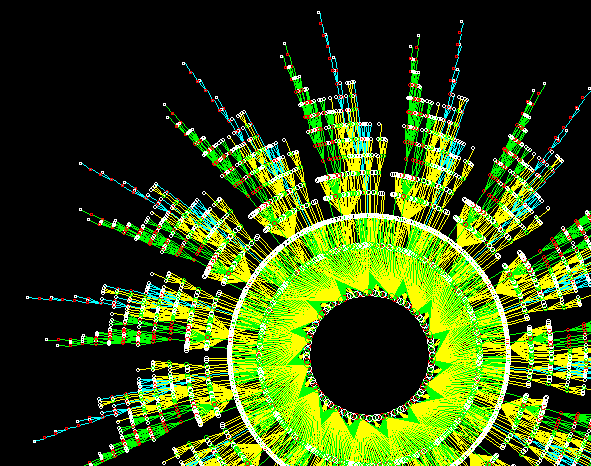The basin of attraction shown on the front cover,
n=5 rule (dec) 54461424, L=15, seed singleton.

 Seven pages of Color Plates were included in the Atlas, showing 28 examples of basins of attraction. These are reproduced below. These images differ slightly from the originals in the Atlas, as they have been reconstructed with DDLab, not with the original DOS software. This was included on diskette with the Atlas, which also contains the Operating Instructions. This original software is available here. Each image is labeled as in the Atlas, where n = neighbourhood size, and L = system size (as opposed to the current labeling convention, k = neighbourhood size, n = system size). Click on each image to see it full size.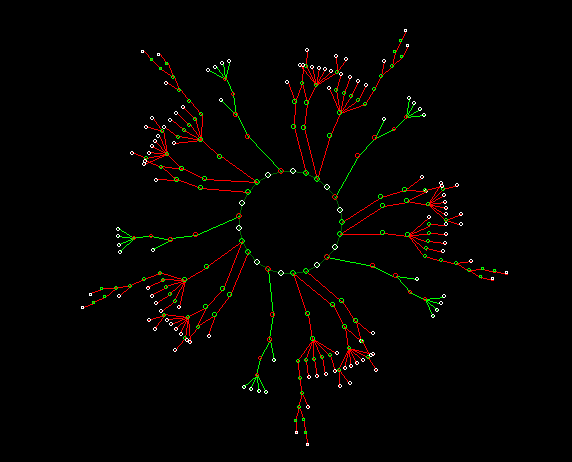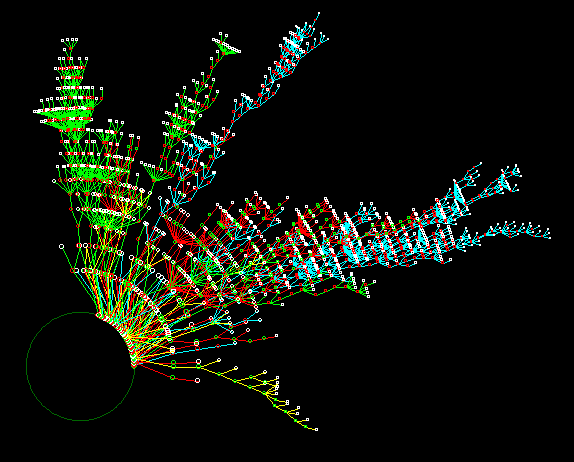n=3 rule 193, L=10, seed singleton n=3 rule 193, L=15, seed 110011000001101 Equivalent transient trees suppressed.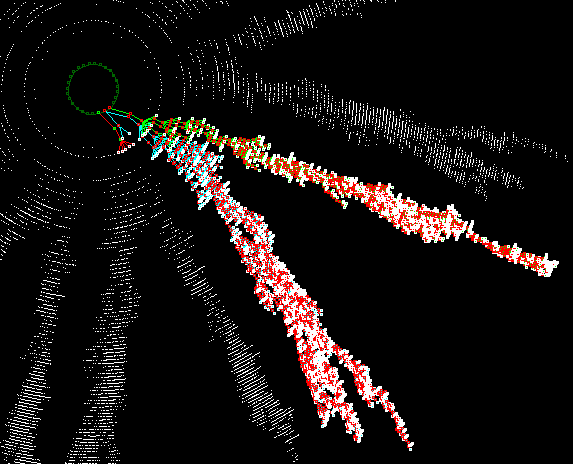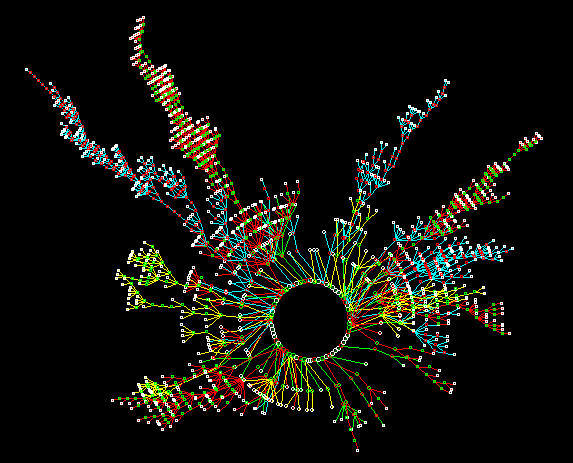n=3 rule 193, L=18, seed 011010001110000010 Equivalent transient trees suppressed. n=3 rule 41 (mutant 1), L=15, seed 110011000001101 this is the n=5 rule 8c c8 0c c3.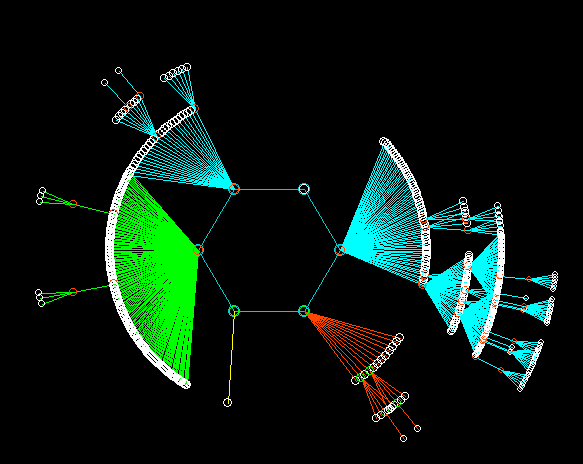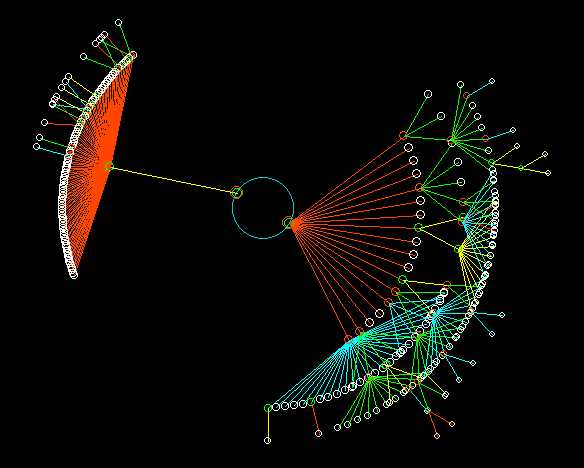n=3 rule 18, L=18, seed 101000000101000000 n=3 rule 33, L=16, seed 0111111111100000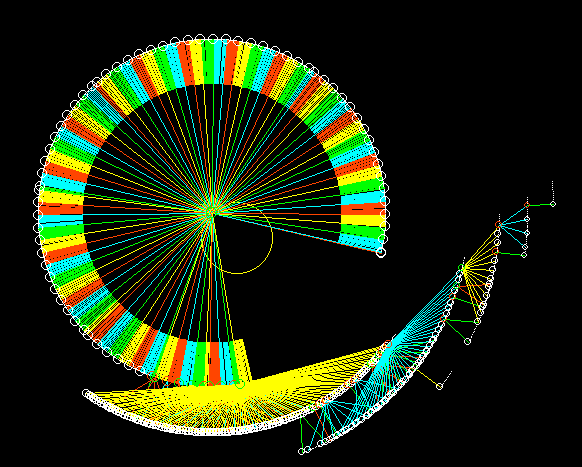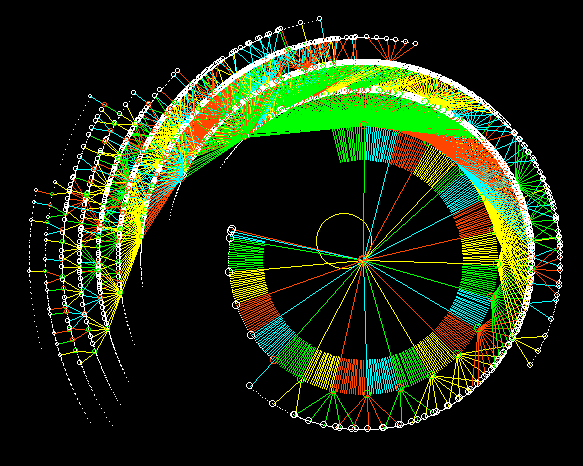n=3 rule 251, L=12, seed singleton Equivalent transient trees suppressed. n=3 rule 249, L=15, seed all 0s Equivalent transient trees suppressed.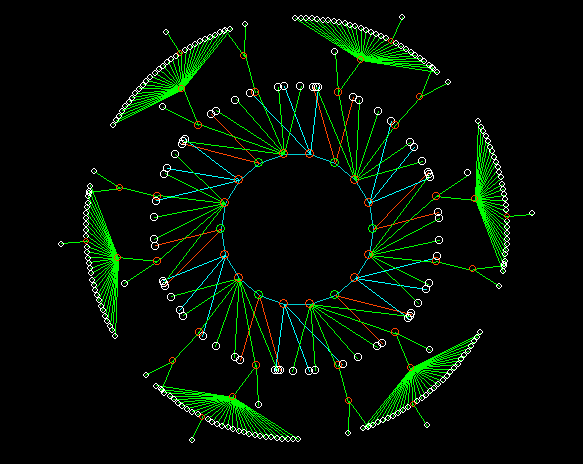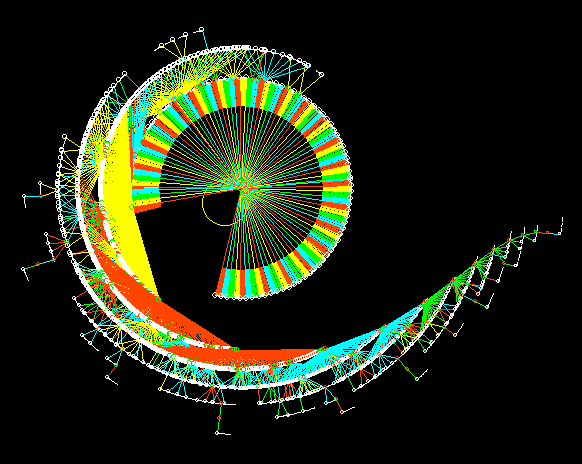n=3 rule 9, L=12, seed 010000010001 n=3 rule 250, L=15, seed all 1s Equivalent transient trees suppressed.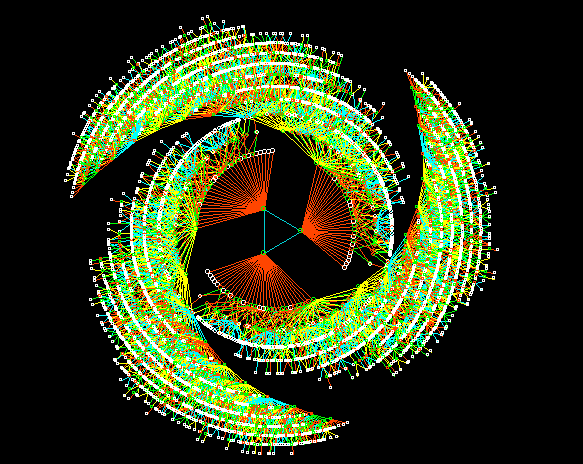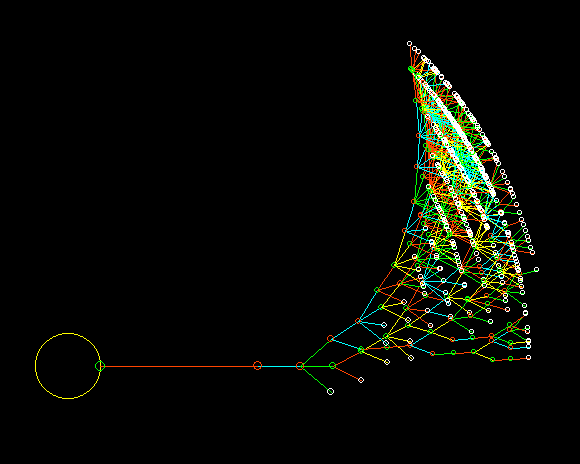n=3 rule 58, L=15, seed 01011011011011 n=3 rule 228, L=15, seed singleton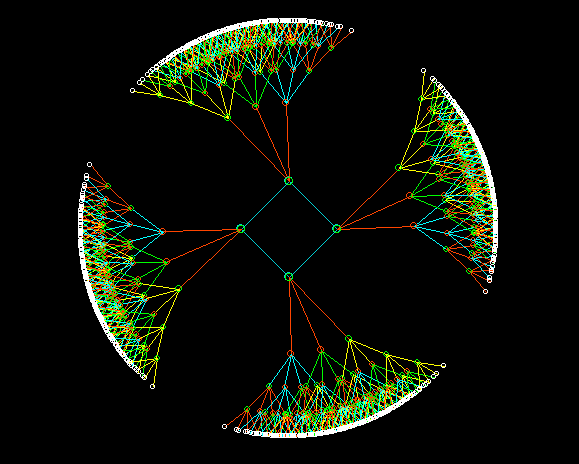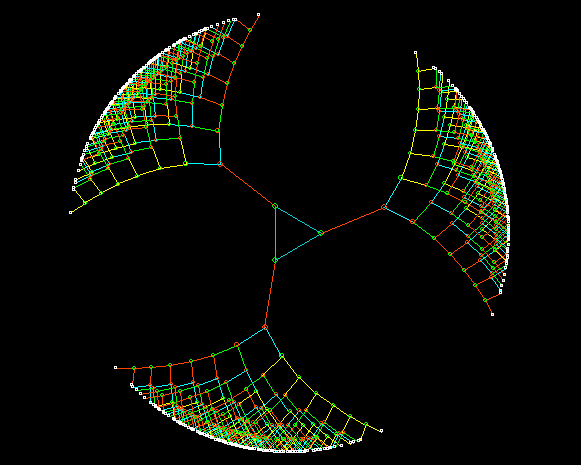n=3 rule 90, L=24, seed 100000000000100000000000 n=3 rule 60, L=24, seed 011011011011011011011011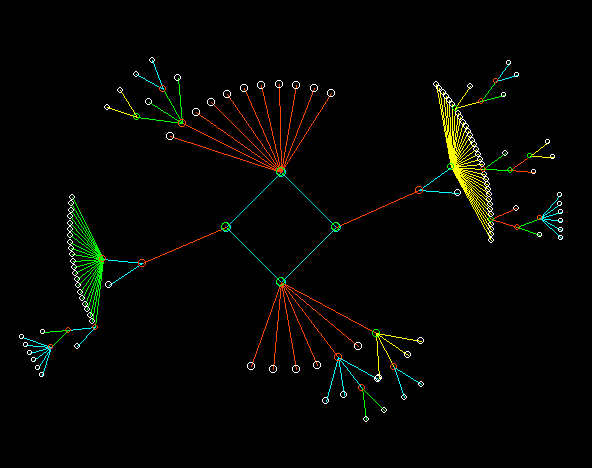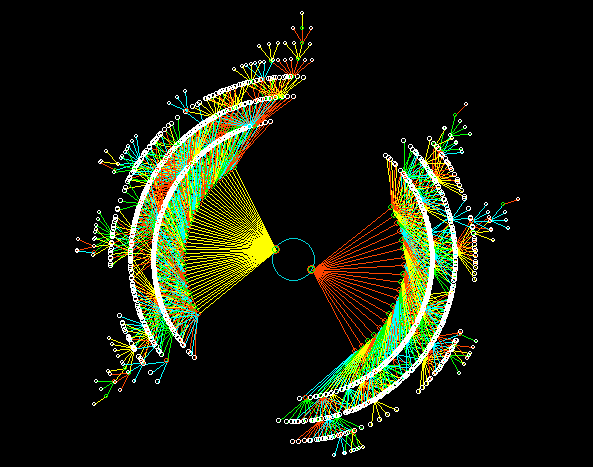n=3 rule 126, L=11, seed singleton. n=3 rule 51 (mutant 1), L=31, seed singleton. this is the n=5 rule 8f 0f 0f 0f.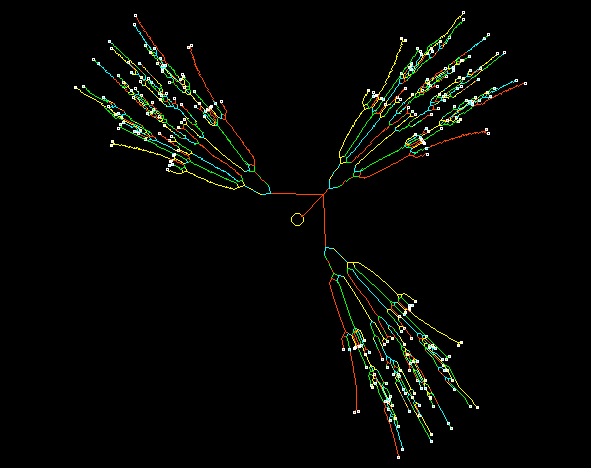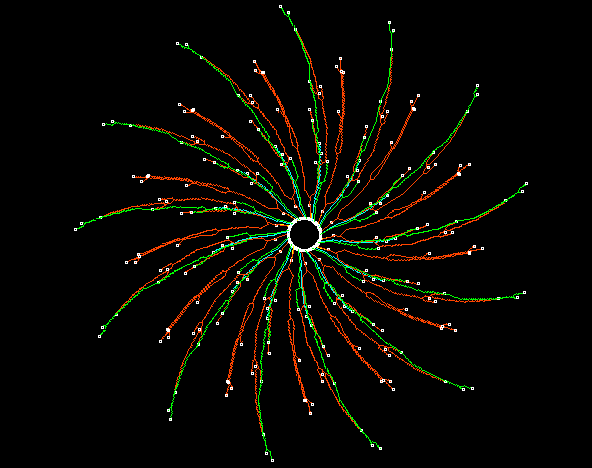n=3 rule 225, L=12, seed all 0s. n=3 rule 225, L=13, seed 2769.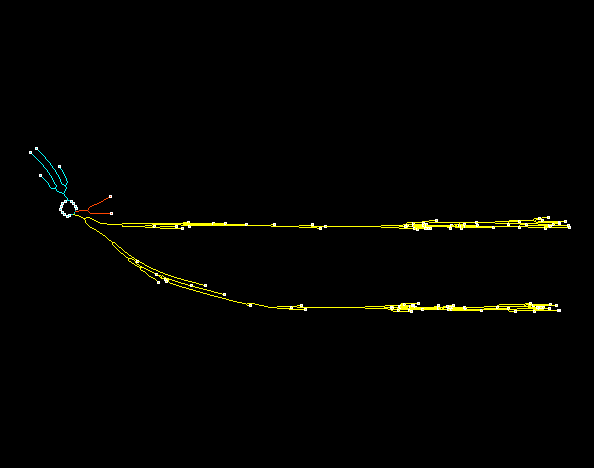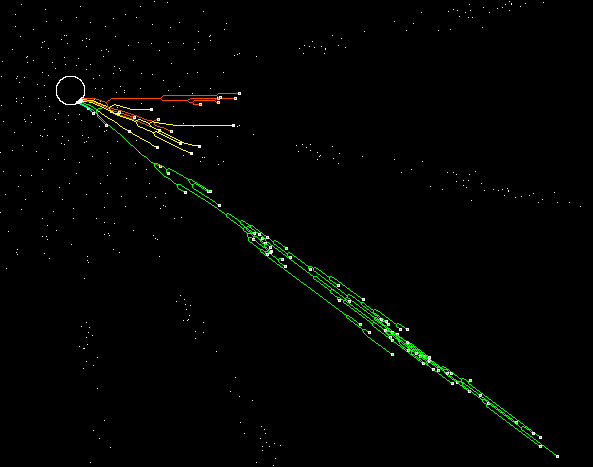n=3 rule 225, L=16, seed 1000000010000000 n=3 rule 30, L=15, seed 28096 Equivalent transient trees suppressed.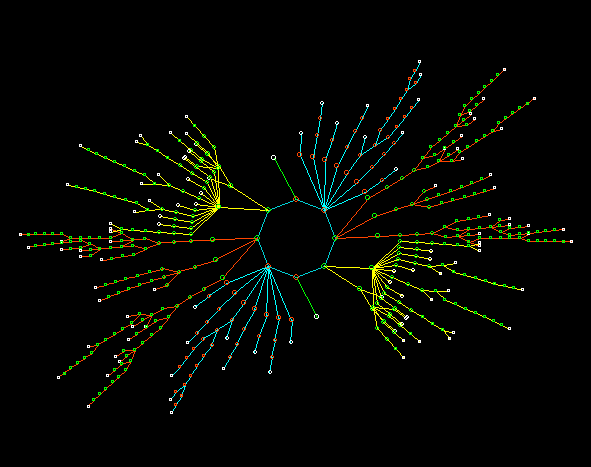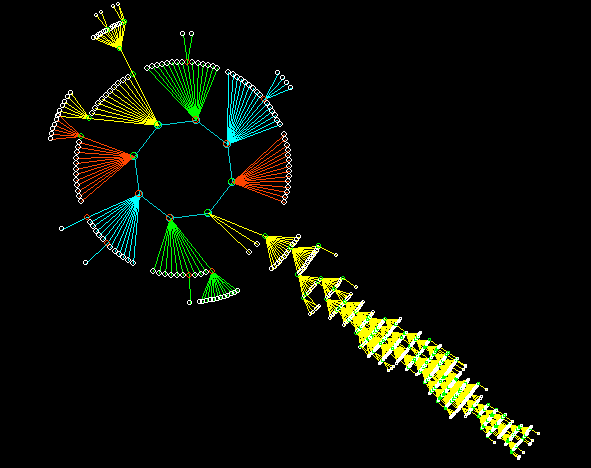n=3 code 10, L=16, seed singleton. n=3 code 10, L=15, seed singleton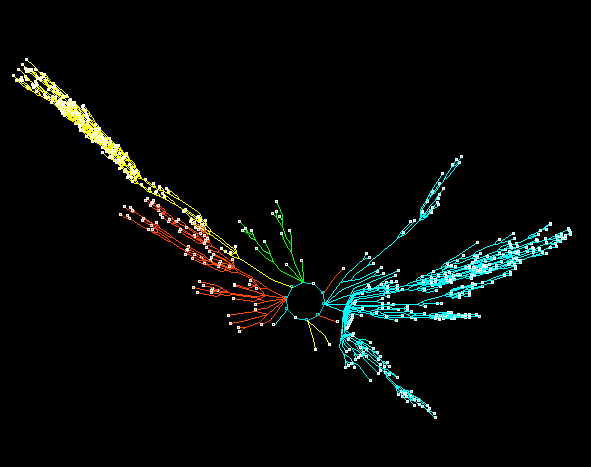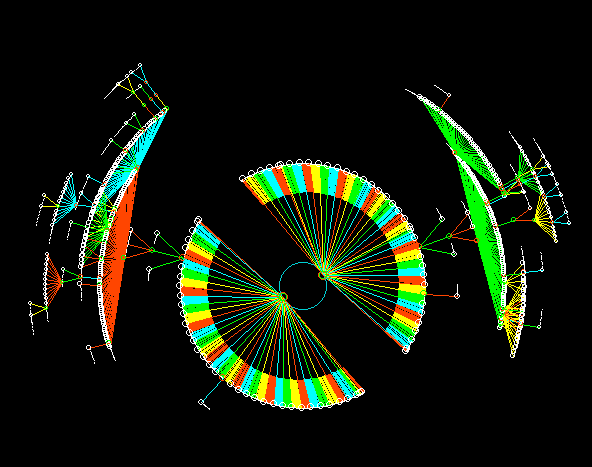n=5 code 10, L=19, seed singleton. n=5 code 25, L=15, seed all 0s Equivalent transient trees suppressed.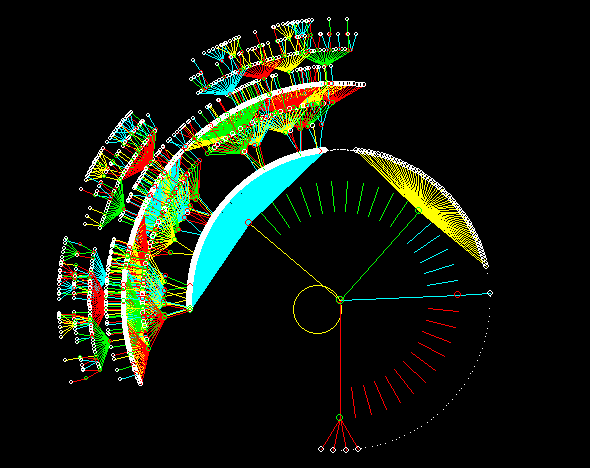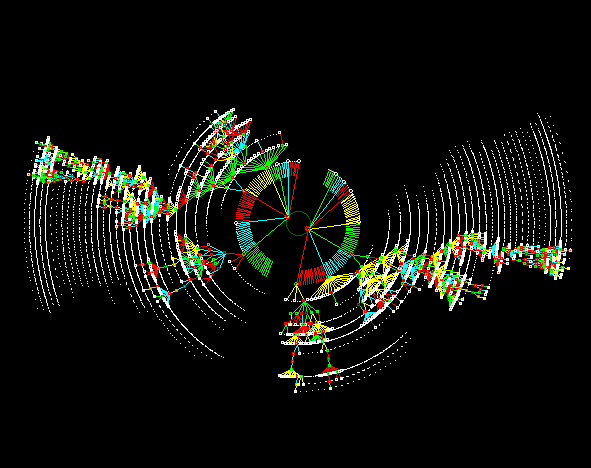n=5 code 14, L=12, seed all 0s Equivalent transient trees suppressed. n=5 code 11, L=15, seed all 0s Equivalent transient trees suppressed.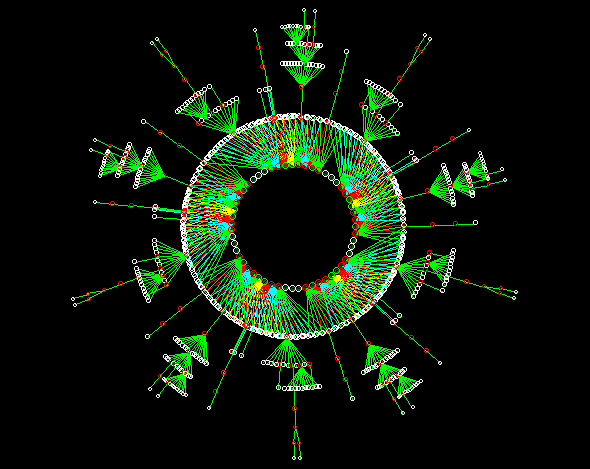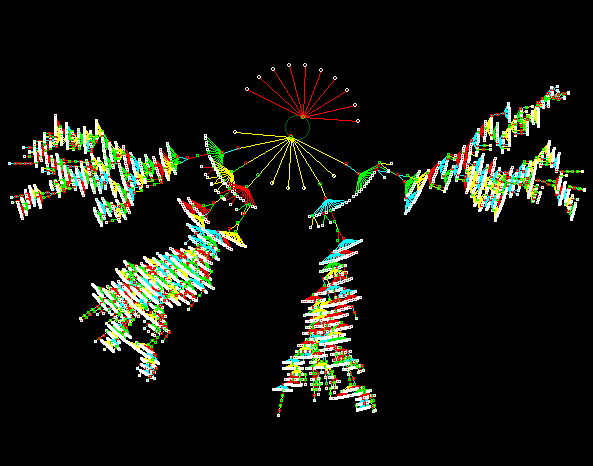n=5 code 53, L=15, seed 101111101101100 n=5 code 53, L=15, seed singleton

back to the start of Color Plates
back to The Global Dynamics of Cellular Automata